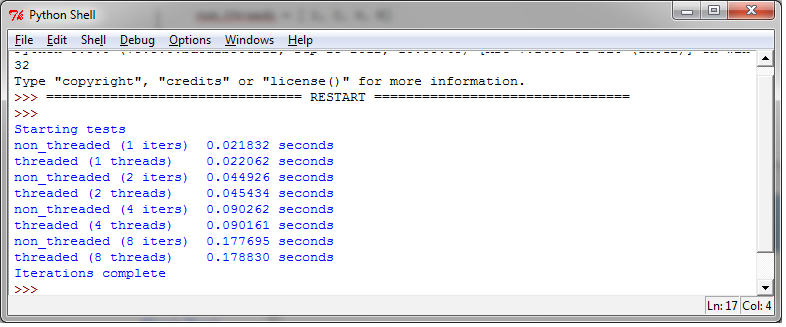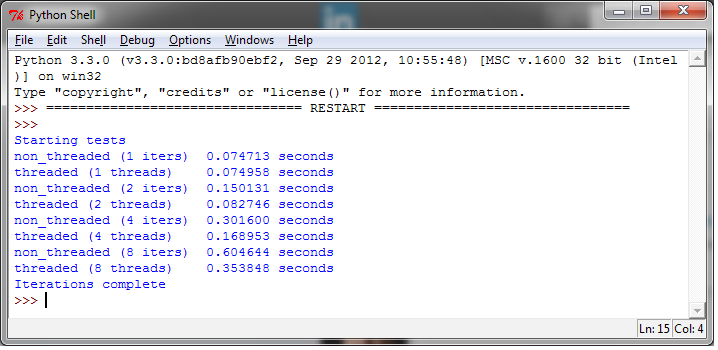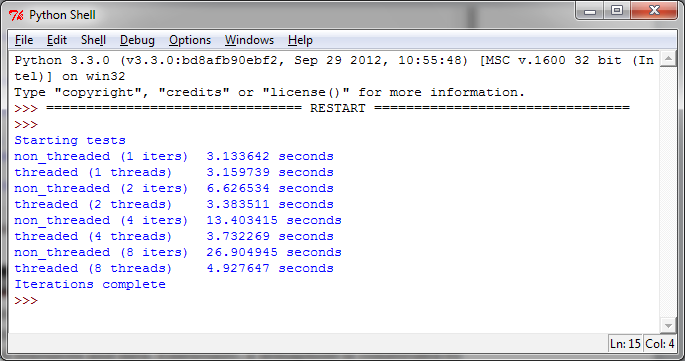# 13. 评估多线程应用的性能¶

## 13.1. 如何做…¶

```from threading import Thread

def run(self):
function_to_run()

def run(self):
function_to_run()

funcs = []
for i in range(int(num_iter)):
for i in funcs:
i.run()

funcs = []
for i in funcs:
i.start()
for i in funcs:
i.join()

def function_to_run():
pass

def show_results(func_name, results):
print("%-23s %4.6f seconds" % (func_name, results))

if __name__ == "__main__":
import sys
from timeit import Timer
repeat = 100
number = 1
num_threads = [1, 2, 4, 8]
print('Starting tests')
best_result = min(t.repeat(repeat=repeat, number=number))
show_results("non_threaded (%s iters)" % i, best_result)
best_result = min(t.repeat(repeat=repeat, number=number))
print('Iterations complete')
```

## 13.2. 讨论¶

### 第一次测试¶

```def function_to_run():
pass
```### 第二次测试¶

```def function_to_run():
a, b = 0, 1
for i in range(10000):
a, b = b, a + b
```### 第三次测试¶

```def function_to_run():
fh=open("C:\\CookBookFileExamples\\test.dat","rb")
size = 1024
for i in range(1000):
```### 第四次测试¶

```def function_to_run():
import urllib.request
for i in range(10):
with urllib.request.urlopen("https://www.packtpub.com/")as f: